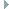Murl::Graph::Transform Class Reference

The Transform node class. More...

`#include "murl_graph_transform.h"`

Inheritance diagram for Murl::Graph::Transform:Public Types inherited from Murl::IFactoryObject< INode >
using ClassInfoArray = Array< const ClassInfo * >
Definition of an array of ClassInfo objects.Public Member Functions inherited from Murl::IFactoryObject< INode >
~IFactoryObject () override
The destructor.

virtual const ClassInfo * GetObjectClassInfo () const=0
Get the object instance's class info, if present. More...

virtual void ResetObjectProperties ()=0
Reset the object instance's properties to their default values.Static Public Member Functions inherited from Murl::IFactoryObject< INode >
static const PropertyInfoGetPropertyInfo ()
Get the class' property info struct. More...

static const AttributeInfoGetAttributeInfo ()
Get the class' attribute info struct. More...

static void ResetProperties (IFactoryObject< INode > *object)
Reset an object instance's properties to their default values. More...

## Detailed Description

The Transform node class.

## XML Elements

XML Graph Node Tag:
XML Graph Node Attributes:

depthOrder="0" [SInt32]

The depth order. See Murl::Graph::ITransformable::SetDepthOrder().

posX="0.0" [Real]

The translation X component. See Murl::Graph::ITransformable::SetPositionX().

posY="0.0" [Real]

The translation Y component. See Murl::Graph::ITransformable::SetPositionY().

posZ="0.0" [Real]

The translation Z component. See Murl::Graph::ITransformable::SetPositionZ().

angleX="0.0" [Real]

The x-axis angle. The angle value must be given in degrees with unit suffix "d" or "deg" or radians with unit suffix "r" or "rad". See Murl::Graph::ITransformable::SetRotationXYZ().

angleY="0.0" [Real]

The y-axis angle. The angle value must be given in degrees with unit suffix "d" or "deg" or radians with unit suffix "r" or "rad". See Murl::Graph::ITransformable::SetRotationXYZ().

angleZ="0.0" [Real]

The z-axis angle. The angle value must be given in degrees with unit suffix "d" or "deg" or radians with unit suffix "r" or "rad". See Murl::Graph::ITransformable::SetRotationXYZ().

rotationOrder="ZYX" [IEnums::RotationOrder]

The axis rotation order. See Murl::Graph::ITransformable::SetRotationXYZ().

axisX="0.0" [Real]

The rotation axis' X component. See Murl::Graph::ITransformable::SetRotation(const Vector& axis, Real angle).

axisY="0.0" [Real]

The rotation axis' Y component. See Murl::Graph::ITransformable::SetRotation(const Vector& axis, Real angle).

axisZ="1.0" [Real]

The rotation axis' Z component. See Murl::Graph::ITransformable::SetRotation(const Vector& axis, Real angle).

angle="0.0" [Real]

The rotation axis angle. The angle value must be given in degrees with unit suffix "d" or "deg" or radians with unit suffix "r" or "rad". If this attribute is set to a non-zero value the angleX/angleY/angleZ values are ignored. See Murl::Graph::ITransformable::SetRotation(const Vector& axis, Real angle).

xx="1.0" [Real]

Can be used to directly specify the values of the 4x4 transformation matrix (xx is 1st row and 1st column). Values will be overridden if used in combination with posX, angle etc.

xy="0.0" [Real]

Can be used to directly specify the values of the 4x4 transformation matrix (xy is 1st row and 2nd column). Values will be overridden if used in combination with posX, angle etc.

xz="0.0" [Real]

Can be used to directly specify the values of the 4x4 transformation matrix (xz is 1st row and 3rd column). Values will be overridden if used in combination with posX, angle etc.

xw="0.0" [Real]

Can be used to directly specify the values of the 4x4 transformation matrix (xw is 1st row and 4th column). Values will be overridden if used in combination with posX, angle etc.

yx="0.0" [Real]

Can be used to directly specify the values of the 4x4 transformation matrix (yx is 2nd row and 1st column). Values will be overridden if used in combination with posX, angle etc.

yy="1.0" [Real]

Can be used to directly specify the values of the 4x4 transformation matrix (yy is 2nd row and 2nd column). Values will be overridden if used in combination with posX, angle etc.

yz="0.0" [Real]

Can be used to directly specify the values of the 4x4 transformation matrix (yz is 2nd row and 3rd column). Values will be overridden if used in combination with posX, angle etc.

yw="0.0" [Real]

Can be used to directly specify the values of the 4x4 transformation matrix (yw is 2nd row and 4th column). Values will be overridden if used in combination with posX, angle etc.

zx="0.0" [Real]

Can be used to directly specify the values of the 4x4 transformation matrix (zx is 3rd row and 1st column). Values will be overridden if used in combination with posX, angle etc.

zy="0.0" [Real]

Can be used to directly specify the values of the 4x4 transformation matrix (zy is 3rd row and 2nd column). Values will be overridden if used in combination with posX, angle etc.

zz="1.0" [Real]

Can be used to directly specify the values of the 4x4 transformation matrix (zz is 3rd row and 3rd column). Values will be overridden if used in combination with posX, angle etc.

zw="0.0" [Real]

Can be used to directly specify the values of the 4x4 transformation matrix (zw is 3rd row and 4th column). Values will be overridden if used in combination with posX, angle etc.

wx="0.0" [Real]

Can be used to directly specify the values of the 4x4 transformation matrix (wx is 4th row and 1st column). Values will be overridden if used in combination with posX, angle etc.

wy="0.0" [Real]

Can be used to directly specify the values of the 4x4 transformation matrix (wy is 4th row and 2nd column). Values will be overridden if used in combination with posX, angle etc.

wz="0.0" [Real]

Can be used to directly specify the values of the 4x4 transformation matrix (wz is 4th row and 3rd column). Values will be overridden if used in combination with posX, angle etc.

ww="1.0" [Real]

Can be used to directly specify the values of the 4x4 transformation matrix (ww is 4th row and 4th column). Values will be overridden if used in combination with posX, angle etc.

XML Animation Resource Tags:

<DepthOrderKey interpolation="LINEAR"> [SInt32Key]

The animation key acting on Murl::Graph::ITransformable::SetDepthOrder().

<PositionKey interpolation="LINEAR"> [PositionKey]

The animation key acting on Murl::Graph::ITransformable::SetPosition().

<RotationKey interpolation="LINEAR"> [EulerAngleKey]

The animation key acting on Murl::Graph::ITransformable::SetRotationXYZ().

<RotationKey interpolation="LINEAR"> [AxisAngleKey]

The animation key acting on Murl::Graph::ITransformable::SetRotation(const Vector& axis, Real angle).

<RotationKey interpolation="LINEAR"> [QuaternionKey]

The animation key acting on Murl::Graph::ITransformable::SetRotation(const Quaternion& q).

The documentation for this class was generated from the following file:
• murl_graph_transform.h